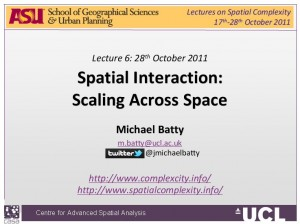# Lecture 6

The sixth lecture introduces our third and last form of scaling: scaling with space, in terms of spatial interaction in two dimensions and density relationships in one. We begin by introducing the traditional population gravity model, show that this is usually specified as being symmetric where potential flows or accessibilities are directly related to the model. In the symmetric case, the flows from i to j are the same as from j to i and the potentials are symmetric. We contrast this with the generic model where origins and destinations are usually asymmetric and we then show how we can build up the family of spatial interaction models by specifying different degrees and types of constraints on their form. These constraints relate to how flows are determined. We then develop one of the best known methods for generating these models – entropy maximising – and so generate the four key models in the family, illustrating how the framework can be used to develop more specific models. We then introduce the basic intepretation of entropy and show how it can be related to probability distributions in space. This we illustrate with the population density models and we show that the usual negative exponential function of travel cost can be replaced with a logarithmic function that then leads to a power law, our baseline models for scaling.Here is the lecture. Click on the Full-text PDF size: 491 Kb or on the adjacent image. The lecture was first given on Friday 28th October and posted the same day. This one was hard work too – so much to try to get over in so short a time. Read it twice and think about it if you haven’t come across this sort of stuff before. The Bibliography page hasn’t yet been updated yet and probably at this rate after the course has finished.